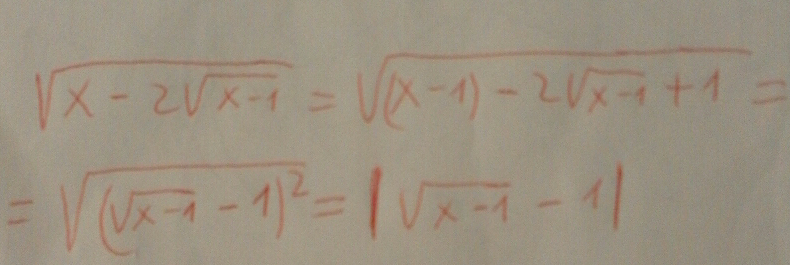# One rule, three different functions.

Jozef Dobos shared this question 2 years ago

I'm trying to solve the equation

sqrt(x-2sqrt(x-1))=sqrt(x-1)-1.

To my surprise, Simplify(sqrt(x-2sqrt(x-1))) gives an unexpected result: sqrt(abs(x-1))-1.1

Simplify will choose one of the branches in this case. For more control you can try:

```Assume(x<2,Simplify(sqrt(x-2sqrt(x-1))))
Assume(x>2,Simplify(sqrt(x-2sqrt(x-1))))```

https://wiki.geogebra.org/e...1

"Assume(x>2,Simplify(sqrt(x-2sqrt(x-1)))) yields sqrt(abs(x - 1)) + 1"

No, this yields sqrt(x - 1) + 1.

How do I know I have to distinguish these two cases when solving my equation?

Compare with

https://www.wolframalpha.co...1

I think the correct answer must be abs(sqrt(x - 1) - 1)1

I'm trying to solve my equation. See attached file.1

I'm trying to solve my equation. See attached file. This is not correct, by mu opinion.

Files: eq.ggb1

Please post the equations here, and what you think the correct answer is11

Sorry, GeoGebra doesn't support equations like this1

WolframAlpha has no problem :)

https://www.wolframalpha.co...1

but Simplify(sqrt(x-2sqrt(x-1))) is not an equation1

Michael,

In this case I expect in GeoGebra something like this:

"Sorry, GeoGebra doesn't support equations like this",

but not "x=d_0^2+1".1